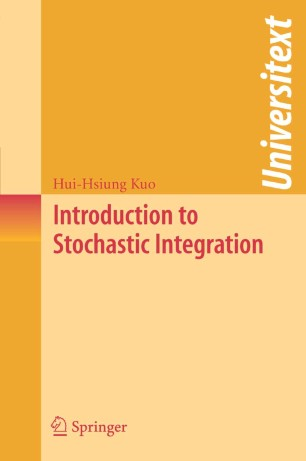Introduction to Stochastic Integration

• Hui-Hsiung KuoTextbook

Part of the Universitext book series (UTX)

1. Front Matter
Pages i-xiii
2. Pages 1-6
3. Pages 7-22
4. Pages 23-36
5. Pages 37-60
6. Pages 61-74
7. Pages 75-92
8. Pages 93-114
9. Pages 115-146
10. Pages 147-184
11. Pages 185-230
12. Pages 231-266
13. Back Matter
Pages 267-278

Introduction

The theory of stochastic integration, also called the Ito calculus, has a large spectrum of applications in virtually every scientific area involving random functions, but it can be a very difficult subject for people without much mathematical background. The Ito calculus was originally motivated by the construction of Markov diffusion processes from infinitesimal generators. Previously, the construction of such processes required several steps, whereas Ito constructed these diffusion processes directly in a single step as the solutions of stochastic integral equations associated with the infinitesimal generators. Moreover, the properties of these diffusion processes can be derived from the stochastic integral equations and the Ito formula. This introductory textbook on stochastic integration provides a concise introduction to the Ito calculus, and covers the following topics:

* Constructions of Brownian motion;

* Stochastic integrals for Brownian motion and martingales;

* The Ito formula;

* Multiple Wiener-Ito integrals;

* Stochastic differential equations;

* Applications to finance, filtering theory, and electric circuits.

The reader should have a background in advanced calculus and elementary probability theory, as well as a basic knowledge of measure theory and Hilbert spaces. Each chapter ends with a variety of exercises designed to help the reader further understand the material.

Hui-Hsiung Kuo is the Nicholson Professor of Mathematics at Louisiana State University. He has delivered lectures on stochastic integration at Louisiana State University, Cheng Kung University, Meijo University, and University of Rome "Tor Vergata," among others. He is also the author of Gaussian Measures in Banach Spaces (Springer 1975), and White Noise Distribution Theory (CRC Press 1996), and a memoir of his childhood growing up in Taiwan, An Arrow Shot into the Sun (Abridge Books 2004).

Keywords

Brownian motion Gaussian measure Martingale Measure Probability theory Stochastic Differential Equations diffusion process linear optimization random function

Authors and affiliations

• Hui-Hsiung Kuo
• 1
1. 1.Department of MathematicsLouisiana State UniversityBaton RougeUSA

Bibliographic information

Industry Sectors
Pharma
Biotechnology
Finance, Business & Banking
Telecommunications
Aerospace